# 形状记忆合金管接头固紧力跌落数值仿真分析Numerical Simulation Analysis on the Drop of Fastening Force of Shape Memory Alloy Pipe Connection

DOI: 10.12677/MOS.2018.73018, PDF, HTML, XML, 下载: 596  浏览: 1,005  国家科技经费支持

Abstract: The drop of the fastening force of the shape memory alloy (SMA) pipe connection will lead to the fall off of the connecting fittings in the process of subsequent use, which will affect the safety and reliability of the whole system. In order to systematically study the drop law of the SMA pipe con-nection fastening force, the nonlinear finite element ABAQUS user-defined material subroutine (UMAT) program is employed to simulate the assembly process of the pipe connection system with different pipe connection wall thickness. The results show that the drop of the fastening force occurs in the middle part of the SMA pipe connection, and with the same wall thickness of SMA pipe connection, the drop of the fastening force is more obvious before cooling down during the assembly process. The contact pressure between the inner wall of the SMA pipe connection and the outer wall of the connected pipe, and the martensite volume fraction of the inner wall of the pipe connection gradually increase with the increase of the wall thickness of the SMA pipe connection; and in the middle part of the inner wall of the SMA pipe connection, the martensite volume fraction of the inner wall of the pipe connection increases to different degrees. The work in this paper can provide the mechanical foundation and technical reference for the optimization design of SMA pipe connection.

1. 引言

2. 理论模型

2.1. SMA本构关系

${\epsilon }_{ij}={\epsilon }_{ij}\left(\xi ,T,{\sigma }_{ij}\right)={\epsilon }_{ij}^{\text{el}}+{\epsilon }_{ij}^{\text{tr}}+{\epsilon }_{ij}^{\text{th}}$ (1)

$\text{d}{\epsilon }_{ij}={S}_{ijkl}\text{d}{\sigma }_{kl}+{\Lambda }_{ij}\text{d}\xi +{\Theta }_{ij}\text{d}T$ (2)

$F\left(\xi \right)=\left(1-\xi \right){F}_{\text{A}}+\xi {F}_{\text{M}}$ (3)

${\Lambda }_{ij}=\left\{\begin{array}{ll}\frac{3H}{2{\sigma }^{\text{eq}}}{\stackrel{˜}{\sigma }}_{ij}\hfill & \stackrel{˙}{\xi }>0\hfill \\ \frac{H}{{\stackrel{¯}{\epsilon }}^{\text{tr}}}{\epsilon }_{ij}^{\text{tr}}\hfill & \stackrel{˙}{\xi }<0\hfill \end{array}$ (4)

${\sigma }^{\text{eq}}=\sqrt{\frac{3}{2}{\stackrel{˜}{\sigma }}_{ij}^{2}}$ (5)

${\stackrel{¯}{\epsilon }}^{\text{tr}}=\sqrt{\frac{2}{3}{\left({\epsilon }_{ij}^{\text{tr}}\right)}^{2}}$ (6)

$\text{d}{\sigma }_{kl}={C}_{ijkl}\left(\xi \right)\left(\text{d}{\epsilon }_{ij}-{\Lambda }_{ij}\text{d}\xi -{\Theta }_{ij}\left(\xi \right)\text{d}T\right)$ (7)

2.2. SMA相变控制方程

$\xi =\frac{1}{2}\mathrm{cos}\left[\frac{\text{π}}{{\sigma }_{\text{ms}}-{\sigma }_{\text{mf}}}\left({\sigma }^{\text{eq}}-{\sigma }_{\text{mf}}\right)\right]+\frac{1}{2}$ (8)

$\left\{\begin{array}{l}{\sigma }_{\text{ms}}={\sigma }_{\text{scr}}+{C}_{\text{M}}\left(T-{M}_{\text{s}}\right)\\ {\sigma }_{\text{mf}}={\sigma }_{\text{fcr}}+{C}_{\text{M}}\left(T-{M}_{\text{f}}\right)\end{array}$ (9)

$\left\{\begin{array}{l}{\sigma }_{\text{as}}={C}_{\text{A}}\left(T-{A}_{\text{s}}\right)\\ {\sigma }_{\text{af}}={C}_{\text{A}}\left(T-{A}_{\text{f}}\right)\end{array}$ (10)

$\xi =\frac{{\xi }_{0}}{2}\left\{\mathrm{cos}\left[\frac{\text{π}}{{A}_{\text{f}}-{A}_{\text{s}}}\left(T-{A}_{\text{s}}-\frac{{\sigma }^{\text{eq}}}{{C}_{\text{A}}}\right)\right]+1\right\}$ (11)

2.3. 物理模型

3. 模拟结果及分析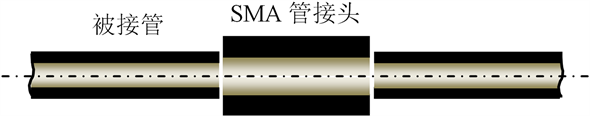Figure 1. SMA pipe connection system assembly profileTable 1. Material parameters of NiTi shape memory alloy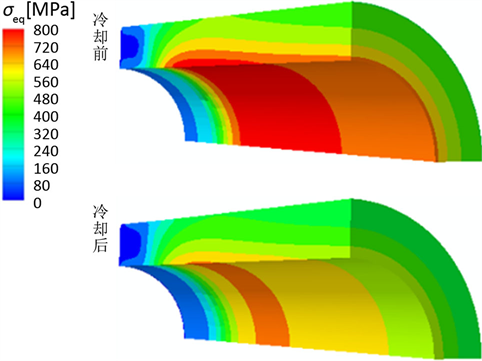Figure 2. Equivalent stress distribution of the connected pipe before and after cooling down

4. 结论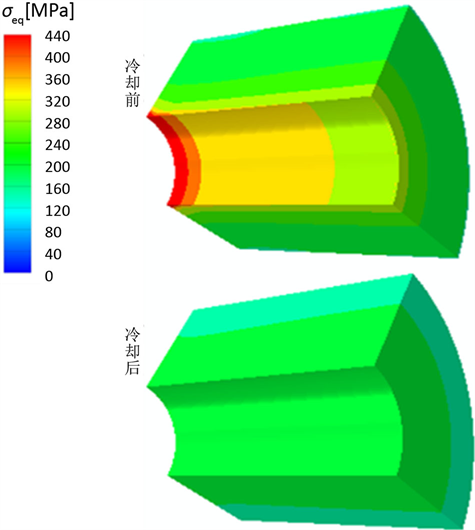Figure 3. Equivalent stress distribution of the pipe connection before and after cooling down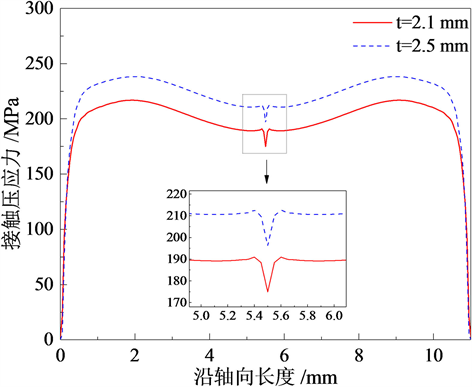Figure 4. Contact pressure distribution curve of inner wall of pipe connection before cooling down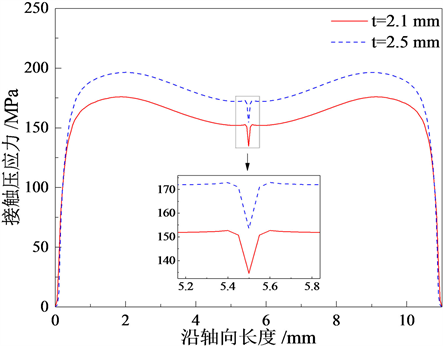Figure 5. Contact pressure distribution curve of inner wall of pipe connection after cooling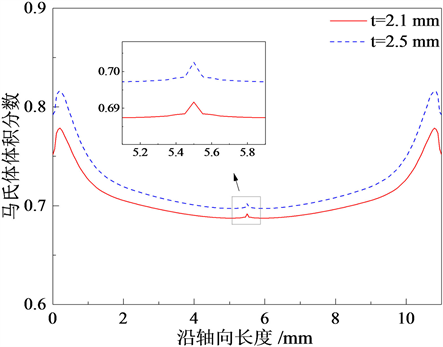Figure 6. Martensite volume fraction distribution curve of inner wall of pipe connection before cooling down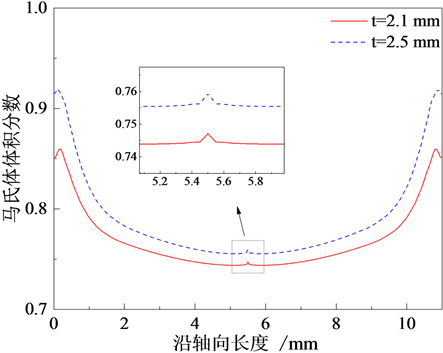Figure 7. Martensite volume fraction distribution curve of inner wall of pipe connection after cooling down

1) 管接头内壁与被接管外壁之间接触压应力在接触中间部位发生跌落；

2) 随着SMA管接头壁厚增大，管接头内壁与被接管接触中间部位的接触压应力以及管接头内壁马氏体体积分数均增大；

3) SMA管接头壁厚较大时，管接头内壁与被接管接触中间部位的接触压应力在整个管接系统装配完成冷却前后均发生不同程度的跌落现象，且在相同壁厚下，冷却后的跌落现象相较于冷却前更加明显；

4) SMA管接头壁厚较大时，管接头内壁与被接管接触中间部位受力情况较为复杂，该部位马氏体体积分数较邻近区域在整个管接系统装配冷却前后均发生不同程度的突然增大。

  Dordoni, E., Meoli, A., Wu, W., et al. (2014) Fatigue Behavior of Nitinol Peripheral Stents: The Role of Plaque Shape Studied with Computational Structural Analyses. Medical Engineering & Physics, 36, 842-849. https://doi.org/10.1016/j.medengphy.2014.03.006  Azaouzi, M., Makradi, A. and Belouettar, S. (2012) Dep-loyment of a Self-Expanding Stent inside an Artery: A Finite Element Analysis. Materials & Design, 41, 410-420. https://doi.org/10.1016/j.matdes.2012.05.019  Hartl, D.J., Lagoudas, D.C. and Calkins, F.T. (2011) Advanced Methods for the Analysis, Design, and Optimization of SMA-Based Aero-Structures. Smart Materials & Structures, 20, Article ID: 094006. https://doi.org/10.1088/0964-1726/20/9/094006  Zhou, B. (2012) A Macroscopic Constitutive Model of Shape Memory Alloy Considering Plasticity. Mechanics of Materials, 48, 71-81. https://doi.org/10.1016/j.mechmat.2012.02.001  Cisse, C., Zaki, W. and Zined, T.B. (2016) A Review of Constitutive Models and Modeling Techniques for Shape Memory Alloys. International Journal of Plasticity, 76, 244-284. https://doi.org/10.1016/j.ijplas.2015.08.006  马彦, 李威. 形状记忆合金管接头结构优化与有限元分析[J]. 东北大学学报(自然科学版), 2013, 34(8): 1166-1170.  严金良, 沈亚鹏, 陈儒. 形状记忆合金管接头空间轴对称有限元分析[J]. 力学学报, 1998, 30(3): 370-378.  韩冬, 刘福顺, 李岩, 等. 不同壁厚的TiNiFe形状记忆合金管接头紧固力的模拟计算[J]. 航空学报, 2006, 27(4): 703-707.  郑雁军, 崔立山, 杨大智. TiNi形状记忆合金回复力模型[J]. 大连理工大学学报, 2000, 40(2): 180-182.  尹向前, 高宝东, 米绪军. TiNiNb形状记忆合金管接头径向压应力的模拟计算[J]. 稀有金属, 2008, 32(5): 579-583.  张慧博, 王健, 金伟, 等. TiNiFe形状记忆合金管接头应力场模拟[J]. 中国有色金属学报, 2010, 20(b10): 510-513.  智友海, 刘永寿, 岳珠峰. 不同载荷下形状记忆合金管接头性能的有限元分析[J]. 机械设计与制造, 2009(2): 4-6.  康泽天, 周博, 薛世峰. 形状记忆合金管接头热机耦合行为的有限元数值模拟[J]. 机械工程学报. http://kns.cnki.net/kcms/detail/11.2187.TH.20180626.1641.026.html  周博, 刘彦菊, 王振清, 等. 形状记忆合金的热力学本构方程 [J]. 固体力学学报, 2011, 32(1): 21-28.  Brison, L.C. (1993) One-Dimension Constitutive Behavior of Shape Memory Alloys: Thermomechanical Derivation with Non-Constant Material Functions and Martensite Internal Variable. Journal of Intelligent Material Systems and Structures, 4, 229-242. https://doi.org/10.1177/1045389X9300400213